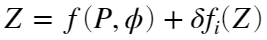## Conversion from water pressure to depth

versión 1.0.0 (1.99 KB) por
Leroy and Parthiot equation is used to the conversion of water pressure to depth. This equation is an alternative to the UNESCO equation.

19 descargas

Ver licencia

UNESCO equation to the conversion of water pressure to depth is widely used to oceanographers around the world. However, the Leroy and Parthiot equation () considers corrective terms, in function of various areas of the world; with this term, the water depth value reaches a better accuracy.
Inputs:
p = water pressure (in MPa, relative to atmospheric pressure).
lat = latitude of the point (in degrees).
D = number of area of applicability.
Output:
z = water depth (in m).
Example:
z = pre2depth(5,[30,45,60],0)
Reference:
Leroy, C. and Parthiot, F. 1998. Depth-pressure relationships in the oceans and seas. J. Acoust. Soc. Am., 103 (3), 1346-1352 p. DOI: 10.1121/1.421275

### Citar como

Gabriel Ruiz-Martinez (2022). Conversion from water pressure to depth (https://www.mathworks.com/matlabcentral/fileexchange/105090-conversion-from-water-pressure-to-depth), MATLAB Central File Exchange. Recuperado .

##### Compatibilidad con la versión de MATLAB
Se creó con R2021b
Compatible con cualquier versión